## Main

We consider two condensates separated in space, and with an initial collisional energy Ecol, which could be in the form of kinetic energy, or potential energy in a magnetic trap. The condensates are then allowed to collide in the z direction. For negligible interactions, the two colliding condensates will interfere like two non-interacting plane waves, forming a standing-wave interference pattern with wavefunction ψcos(k z), where the wavelength of the pattern is λ=2π/k, and k is given by 2k2/2m=Ecol/N, where m is the atomic mass and N is the number of atoms. The density of the pattern is therefore of the form 2 n=|ψ|2cos2(k z). The initial energy Ecol supplies the kinetic energy of the standing wave, proportional to 2ψ/ z2, as well as the negligible interaction energy N μ/4 (see Supplementary Information). However, if Ecolis decreased to become comparable to N μ/4, the form of the fringes will be modified by interactions. It will become energetically favourable to form an array of grey solitons, rather than a cosine-squared pattern2. This soliton formation process is relevant for the operation of an atom interferometer14. The criterion Ecol<N μ/4 can be written as λ>4πξ, where ξ is the healing length, the approximate width of a grey soliton15,16. In other words, as Ecoldecreases, the wavelength of the standing-wave interference pattern increases until the wavelength reaches the size of a soliton. At this point, the fringes will evolve into solitons. A cosine-squared fringe and a soliton are actually very similar in form, as seen in Fig. 1a,b. As Ecol decreases further, the number of solitons will decrease, maintaining conservation of energy3. The lowest collisional energies will result in two grey solitons travelling in opposite directions. Such a low collisional energy is achieved in our experiment by initially separating the two condensates by a barrier with a width of the order of ξ, as shown in Fig. 1c. The energy of this initial configuration relative to the ground state is of the order of Ecol=N μ(ξ/L), where L is the length of the condensate in the z direction. This is comparable to the energy of a grey soliton computed in one dimension, which is of the order of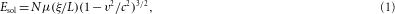where v is the subsonic soliton velocity and c is the speed of sound in the condensate13. Ecol is thus sufficient to create a pair of solitons.

Solitons can also be created by phase engineering17,18,19. There is a phase step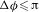across the grey soliton, given by Δφ=2cos−1(v/c). By imposing this phase step, the density profile will evolve into that of a soliton. We can understand this process by considering the current density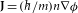. By imposing the phase step, this current density is significant only in the region of the step. This corresponds to a divergence in the flow field,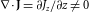. As the condensate obeys the continuity equation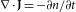, the divergence depletes the density, forming the soliton minimum.

We use density engineering to form solitons, a complementary technique to phase engineering. We impose the density profile, and the phase evolves into that of a soliton. The process is illustrated in Fig. 1d, which shows two solitons separated by a distance d. They are moving in opposite directions, with the corresponding opposite phase steps. Our initial configuration shown in Fig. 1c can be thought of as the d=0 case of Fig. 1d. Our initial configuration thus corresponds to two overlapping, counterpropagating solitons.

In a nearly one-dimensional (1D) condensate, solitons are stable objects20,21. In contrast, in 3D, the stable nonlinear modes are vortex rings, as well as hybrids of solitons and vortex rings22. In previous studies in 3D, grey solitons were seen to decay irreversibly into vortex rings through the snake instability7,8,9,10,11,12, which was originally measured in optical solitons13. We find a very different, oscillatory behaviour, shown in Fig. 2 by a simulation of the 3D Gross–Pitaevskii equation15 (GPE). The soliton evolves into a vortex ring, which subsequently evolves back into a soliton. This process repeats itself many times with a period of 7–8 ms. We call this a ‘periodic soliton/vortex ring’, which involves the repeated revival of a soliton, which is an unstable object in our system. The simulation shows that the periodic soliton/vortex ring is stable, surviving the reflection from the end of the condensate, as well as the subsequent collision in the middle of the condensate. The periodic soliton/vortex ring has a constant phase step (0.7π for our experiment), as shown in Fig. 2j,k.

First, we will explain the evolution of the soliton into a vortex ring in terms of conservation of energy. Owing to the higher density near the centre of the condensate, the soliton moves fastest in this region18. This causes the soliton to curve18, as shown in Fig. 2f. Owing to the curvature, the area of the soliton has increased, corresponding to an increase in the soliton energy. To conserve the energy of the soliton, the increase in area must be compensated by an increase in v, by equation (1). This corresponds to a reduction in the depth of the soliton, as shown in Fig. 1b. The soliton thus moves with ever-increasing speed and decreasing depth, until the density minimum vanishes altogether, leaving a vortex ring, as shown in Fig. 2c,g,i. The appearance of the vortex ring does not violate Kelvin’s theorem (conservation of circulation), because the vortex core can enter and exit the condensate through the nearby boundary23.

The fate of vortex rings in a quantum fluid is a long-standing field of study23,24. In superfluid 4He at non-zero temperatures, it was suggested that the radius of a vortex ring shrinks in time owing to dissipation, until it evolves into a roton. We can understand the very different evolution of our vortex ring into a soliton in terms of conservation of mass. We consider a vortex ring in an infinite homogeneous condensate15,25, as illustrated24 in Fig. 2l. The density is constant in time, maintained by the zero divergence of the flow (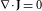). In other words, after the flow traverses the centre of the vortex ring from right to left, it returns to the right outside the vortex ring. In contrast, our vortex ring is close to the edge of the condensate, as shown in Fig. 2c,g,i. The flow lines therefore have no return path to the right side, causing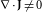. The divergence depletes the density, forming the soliton minimum. This situation is very similar to that of phase engineering of a condensate described above. In fact, the vortex ring has a phase step, as shown in Fig. 2j. The phase step occurs on a length scale Δz of the order of the radius of the vortex ring, which is also the radius R of the condensate. Thus, for creating solitons from vortex rings, R should not be too much larger than ξ. This can also be understood energetically. The periodic soliton/vortex ring requires that the soliton and the vortex ring have the same energy. The ratio of these energies contains a factor of R/ξ, placing an upper limit on R. Using the GPE simulation, we find that the vortex ring evolves into a soliton for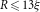, corresponding to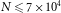for our trap frequencies. Note that it is the radial variation of the condensate rather than the axial variation that causes the evolution.

We carry out density engineering through a narrow, blue-detuned laser barrier, which is adiabatically ramped up in τramp=200 ms to a height U=h(3,000 Hz), creating a Bose–Einstein condensate (BEC) with a density minimum, as shown in Fig. 3a. The potential is then rapidly turned off in 1 ms, resulting in the initial condition illustrated in Fig. 1c. This sequence is indicated by the solid curve in Fig. 3b. As seen in Fig. 3d, the two counterpropagating solitons can be distinguished after 2 ms, which result from the interference between the two condensates in Fig. 3a, colliding with low energy. Figure 3d corresponds to Fig. 1d. Figure 3c shows the simulation at 2 ms with imaging effects, including integration of the density perpendicular to the image, finite resolution and finite depth of field. The experimental images in Fig. 3 agree well with the simulated images. The resolution is taken to be 2 μm, which gives the best visual agreement between the experiment and the simulation. This is larger than the measured 1.2 μm resolution of the imaging system. We attribute this difference to defocusing of the imaging system with its short depth of field.Figure 3: In situ images of the vortex rings and solitons.

After a time τ=5 ms, we obtain in situ images of the pair of counterpropagating vortex rings of the periodic soliton/vortex ring, as shown in Fig. 3g. In the image, the two vortex rings are seen as weak density minima. The weak density minima are also seen in Fig. 3h, which shows the profile along the central axis in Fig. 3g. Owing to the shape of a vortex ring, the density minima should be weak along this central axis. After each of the two vortex rings has evolved back into a soliton, we image the soliton stage at τ=9 ms, as shown in Fig. 3j. Two strong, slit-like solitons are seen. Figure 3k shows the greatly increased contrast along the central axis in the soliton stage, relative to the vortex ring stage in Fig. 3h. This increase in contrast is evidence for the evolution of the vortex ring into a soliton. We find that the solitons are more visible for the small atom number shown in the figure.

To measure the speed of the periodic soliton/vortex ring, we observe its position as a function of time, as shown in the integrated profiles in Fig. 4a, and the filled circles in Fig. 4b. We see that the speed (the slope) is approximately constant in time, and is therefore the same for the soliton stage and the vortex ring stage. The speed is observed to be v=0.66±0.08 mm s−1, for N=3×104. This speed is of the same order of magnitude as the speed v≈(/2m R)ln(1.59R/ξ)≈0.3 mm s−1 predicted for a vortex ring in an infinite, homogeneous condensate15,23,24. Excellent agreement between experiment and simulation is seen throughout Fig. 4.

The speed of a grey soliton is less than c, so it is interesting to compare the speed of the periodic soliton/vortex ring with c. We measure c using the technique of ref. 26. Specifically, we rapidly turn on the barrier in 100 μs and leave it on as indicated by the dashed curve in Fig. 3b, creating two counterpropagating sound pulses, as shown after 5 ms in Fig. 4g. The profiles in Fig. 4c and the open circles in Fig. 4b show the position of the sound pulses as a function of time, giving c=1.24±0.07 mm s−1, which agrees with the theoretical value27 of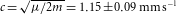. We thus find that the periodic soliton/vortex ring moves slower than the speed of sound, at v=0.53c. This subsonic motion is evident in Fig. 4e,g, in that the two arrows are closer together for the vortex rings than for the sound pulses.